Subject:
Geometry
Material Type:
Lesson Plan
Level:
Middle School
7
Provider:
Pearson
Tags:
• Buildings
• Concepts
• Models
• Prisms
Language:
English
Media Formats:
Text/HTML

# Gallery Problems Exercise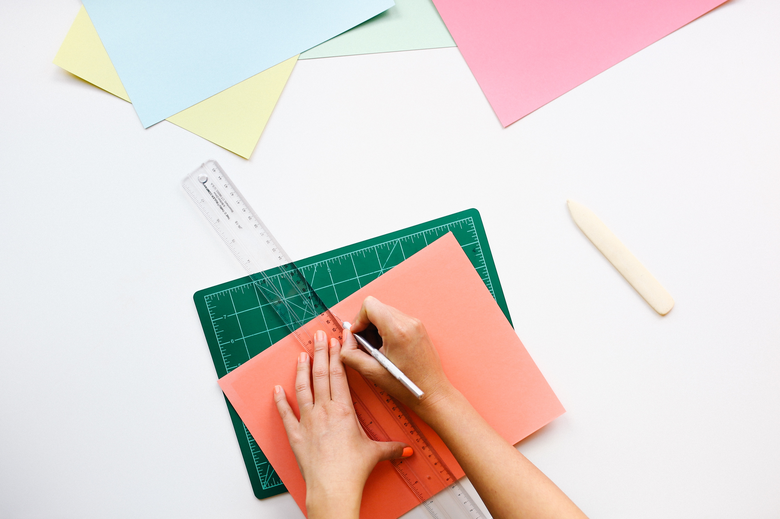# Gallery 2

Allow students who have a clear understanding of the content thus far in the unit to work on Gallery problems of their choosing. You can then use this time to provide additional help to students who need to review the unit’s concepts or have fallen behind on work.

# Gallery Overview

This task gives students an opportunity to further explore figures that have been intersected by a plane. The task also allows students to revisit scale and think about the net of a sliced prism.

Sketch Three
This task extends students’ knowledge of nets as they think about surfaces that are triangular and won’t line up parallel. Students may need to use a protractor to keep the angles of the sides consistent.

Partial Cube Net
This task provides students with further experience in thinking about the revealed surface in a sliced prism, constructing a more complex net, and estimating area based on area formulas and measuring.

Round Prisms
This task extends students’ knowledge of prism measurement to cylinders, which are really no different. Students will see that the only difference is that the base is circular, and they know how to find the circumference (perimeter) and area.

Project Work Time
Students may use a Gallery day to work on their projects and get help if needed.

Cube Volume and Nets
Using the 2-D/3-D tool or the parallelogram cubes, students create a solid made of cubes. Using the 2-D views as a guide, they make a net for the figure and find its surface area. Students are challenged to make the net with one piece of paper.

Same Surface Area, Different Volume
Students create two solids with the same surface area but very different volumes. They that surface areas are the same by drawing the 2-D views.

Tree House 2
This task gives students further practice making a scale drawing and thinking about the net of a solid. Students should also realize that the plans for a building are the 2-D views of the building and are similar to a net.

When a figure is redrawn at a larger scale, the side lengths increase by the factor of the scale (if the scale doubles the size, the side lengths double also). But, does the area increase the same way? Students explore a dynamic sketch and see how area changes when the scale changes.

This task gives students an opportunity to further explore figures that have been intersected by a plane. The task also allows students to revisit scale and think about the net of a sliced prism.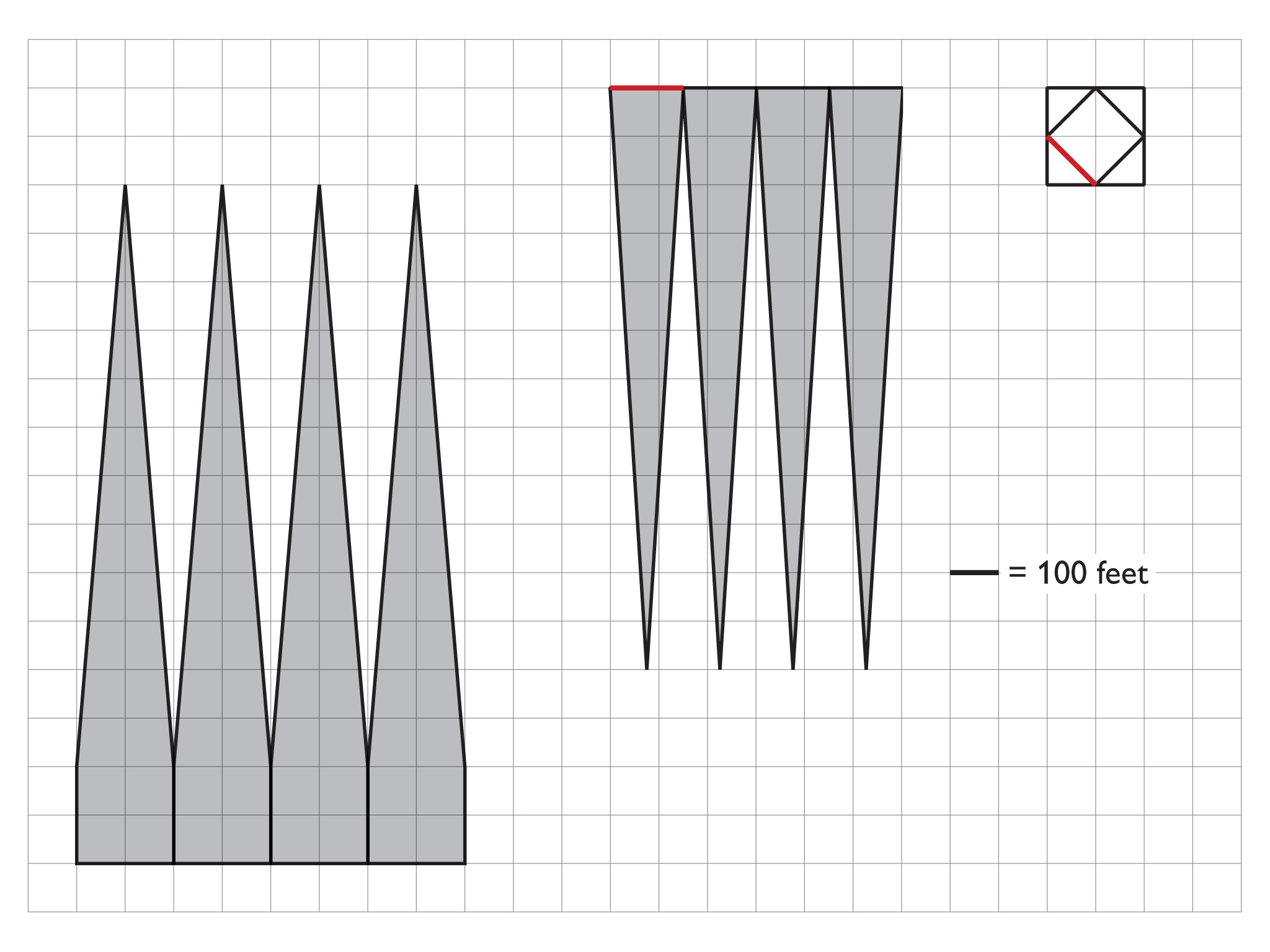1. a. Answers will vary, but the net is probably easier to make in parts, especially because the triangles are slightly longer than the height of the building, since they slope.
b. 1 in. = 100 ft
c. The triangles with their base at the top have a base as wide as the diagonal (about 1.4 square lengths or 140 ft) and a height of about 1,200 ft. The triangles where the bases are located at the bottom of the building have a base of 200 ft and a height of 1,200 ft.
2. Students will cut out the net and construct the model

3. The approximate surface area can be calculated as follows:

The base area is 160,000 ft2, since each side is 200 ft • 200 ft.

The four triangles that meet the square base have a base of 1,2000 ft:

("total height" ≈ 1,400 ft and "base height" ≈ 200 ft, so 1,400 ft − 200 ft = 1,200 ft)

$\left[\frac{\left(200\text{\hspace{0.17em}}\text{ft}\cdot 1,200\text{\hspace{0.17em}}\text{ft}\right)}{2}\right]4=\frac{240,000\text{\hspace{0.17em}}{\text{ft}}^{2}}{2}\cdot 4=480,000\text{\hspace{0.17em}}{\text{ft}}^{2}$

The smaller four triangles have a base of about 140 ft and are also about 1,200 ft in height.

$\left[\frac{\left(140\text{}\text{ft}\cdot 1,200\text{}\text{ft}\right)}{2}\right]4=\frac{168,000\text{}{\text{ft}}^{2}}{2}\cdot 4=336,000\text{}{\text{ft}}^{2}$

Total: $160,000\text{}{\text{ft}}^{2}+480,000\text{}{\text{ft}}^{2}+336,000\text{}{\text{ft}}^{2}=976,000\text{}{\text{ft}}^{2}\approx 1\text{}{\text{million ft}}^{2}$

## Work Time

Here are some interesting facts about the One World Trade Center Building:

• It is 1386 ft tall.
• The base is a 200 ft square.
• The building is a cube up to 200 ft.
• The building is based on a square prism but have diagonal slices out the corners, forming triangle faces.
• Each side is an isosceles triangle and there are two sets of 4 congruent triangles.

1. Use the given information and research more facts about the One World Trade Center Building.
a. Draw a net for the building on 1-in grid paper.
b. How many feet should each inch represent?
c. What are the dimensions of the triangle faces?

2. Cut out the net and construct the model.

3. Estimate the surface area of the building.

# Sketch Three

This task extends students' knowledge of nets as they think about surfaces that are triangular and won't line up parallel. Students may need to use a protractor to keep the angles of the sides consistent.

1. There are several possibilities, considering also that the triangle height can vary from one net to another. The most obvious net is similar to the tree house net or a box with no lid: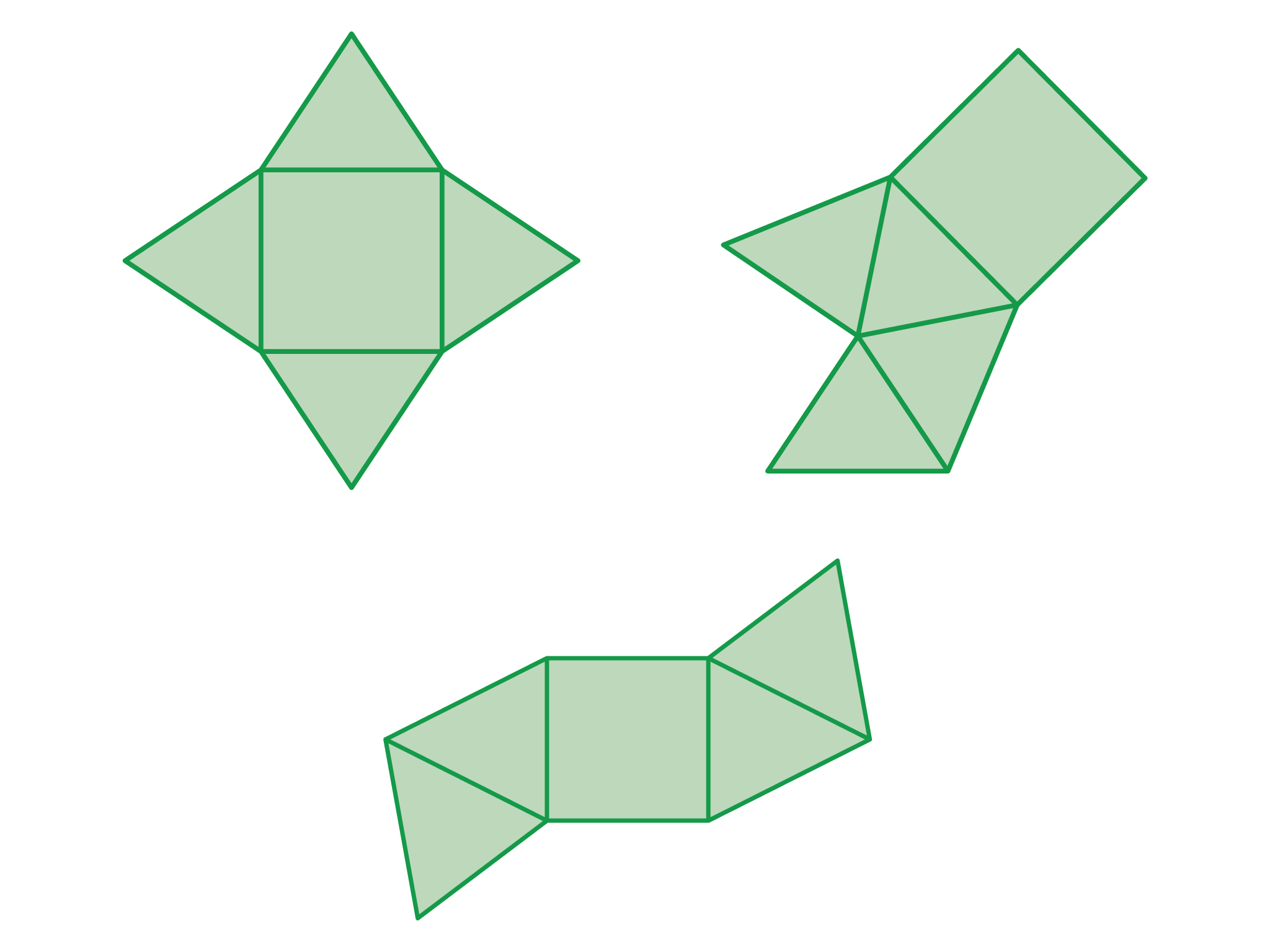2. Students should see that there is no maximum height for the sides, but there is a minimum. The sides must be taller than half the base width (4 cm in this case), or the sides will fold in flat. As the height of the sides increases, the pyramid gets taller.
3. Answers will vary. If students use a whole number measurement for the height, the calculations will be simpler.

# Sketch Three

You will be drawing three different nets in this activity that can make the square pyramid shown in the picture. The pyramid has a square base with 8 cm sides.

1. Draw 3 different nets on 1-cm grid paper that can be folded into the square pyramid in the picture. The height of the pyramid is not shown, so you can decide how tall you can make your pyramid.
2. What is the maximum and minimum height that the pyramid could be? What happens to the pyramid when the height of each of the sides increases?
3. Cut out your pyramids, and assemble them. What is the surface area and volume for each of your pyramids?

As you are doing this activity, ask yourself the following questions to help guide you through:

• Which edges are shared in each of your nets?
• What do the triangular sides have in common?
• How does the shape of the triangle affect the height of the pyramid?
• What kinds of triangles can you use when making your nets?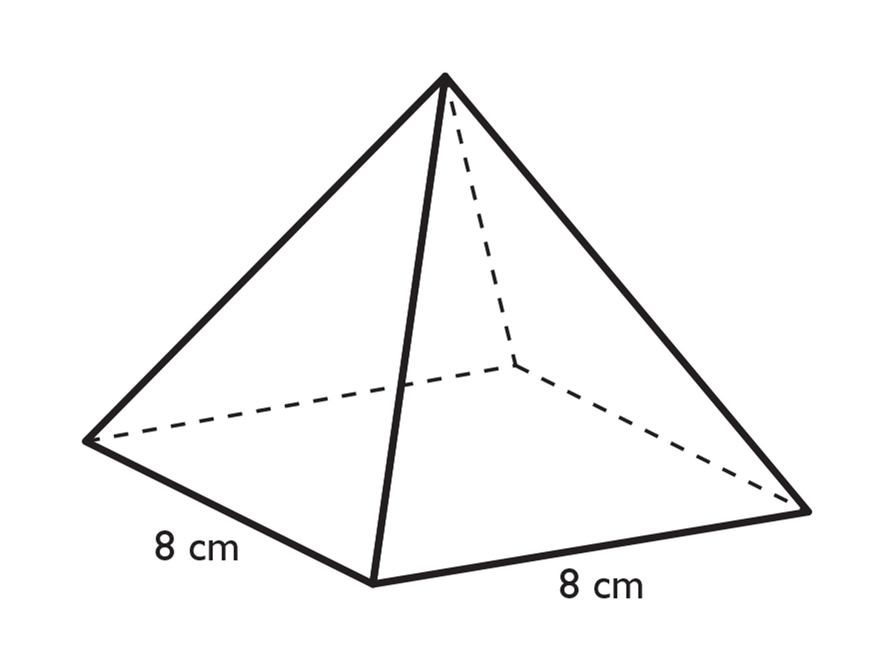# Partial Cube Net

This task provides students with further experience in thinking about the revealed surface in a sliced prism, constructing a more complex net and all that that involves, and estimating area based on area formulas and measuring.

1. Answers will vary, but students will begin with a cube net and go from there. Here is an example: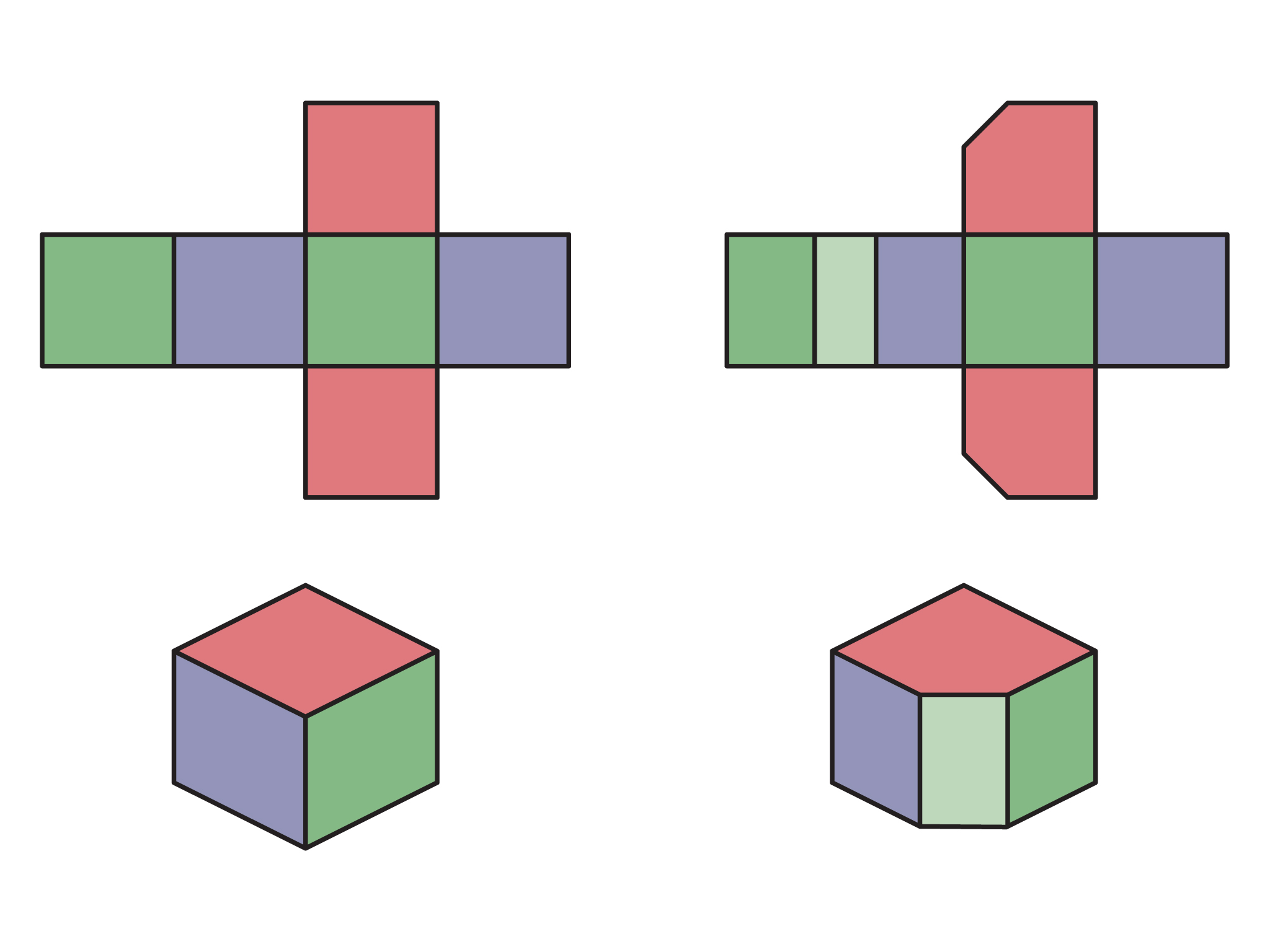2. Answers will vary, but at a minimum three sides will be affected (if a corner is sliced off), and all sides could be affected.
3. Answers will vary. The surface area won’t be too difficult (depending on the cut) because students will have the net to work with. A strategy for the volume would be to start with the volume of the cube and then find the volume of the missing piece, subtracting the second from the first.

# Partial Cube Net

1. Make a net for a cube. Then, picture that the cube has been sliced in some way (but not parallel to a side). How will the net change?
2. Adjust the net to include the revealed surface and the change in the sides. How many sides will the slice affect? Experiment with the net, thinking about which edges will be shared.
3. Construct the figure and find the surface area and estimate the volume.

# Round Prisms

This task extends students' knowledge of prism measurement to cylinders, which are really no different. Students will see that the only difference is that the base is circular, and they know how to find the circumference (perimeter) and area.

1. Answers will vary, but should look very much like this: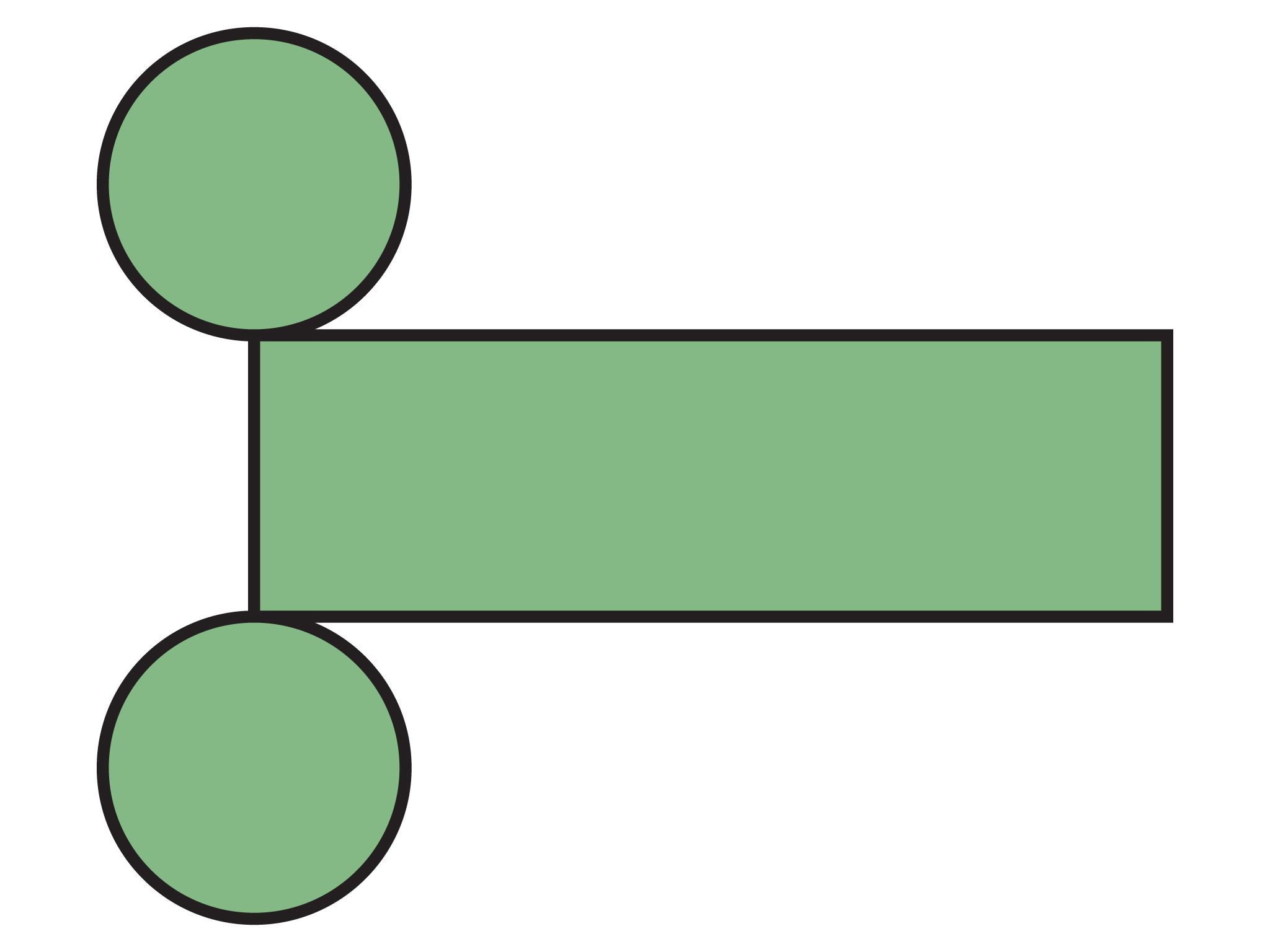Students need to realize (or they will once they begin working on the net) that the length of the lateral surface is the circumference of the circle, which is often longer than students realize.

2. Answers will vary. The surface area is the area of the two bases plus the lateral surface:

$A=2\pi {r}^{2}+\pi dh$

The volume is the area of the base ($\pi {r}^{2}$) multiplied by height:

$V=\pi {r}^{2}h$

3. Answers will vary, but students just need to make the height the same as the circumference. A simple example is the tennis ball can with 3 balls. Its height is 3d, and the circumference (lateral surface length) is $\pi d$. This will create a lateral area that is approximately a square.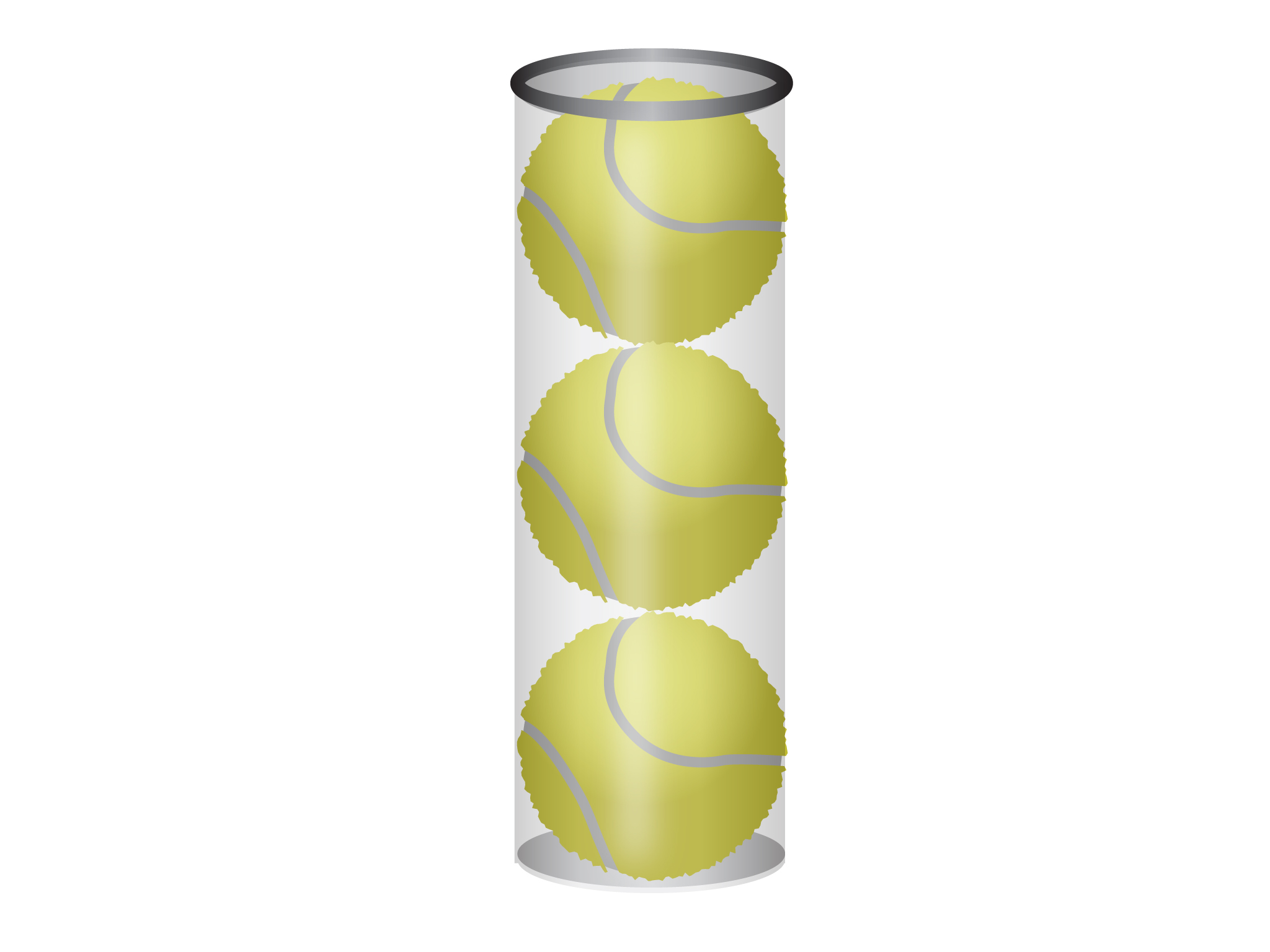# Round Prisms

If a prism has a round base it is called a cylinder. What will the net of a cylinder look like? How will it be similar to a prism with a polygon base?

You know how to find the surface area and volume of a right prism, and the same rules will apply here. Use your knowledge of prisms and circles to do the following:

1. Decide on a height and radius for your cylinder. On grid paper, make a net for your cylinder, and construct it.
2. Find the surface area and volume.
3. If you have time, make a second cylinder with a square lateral surface.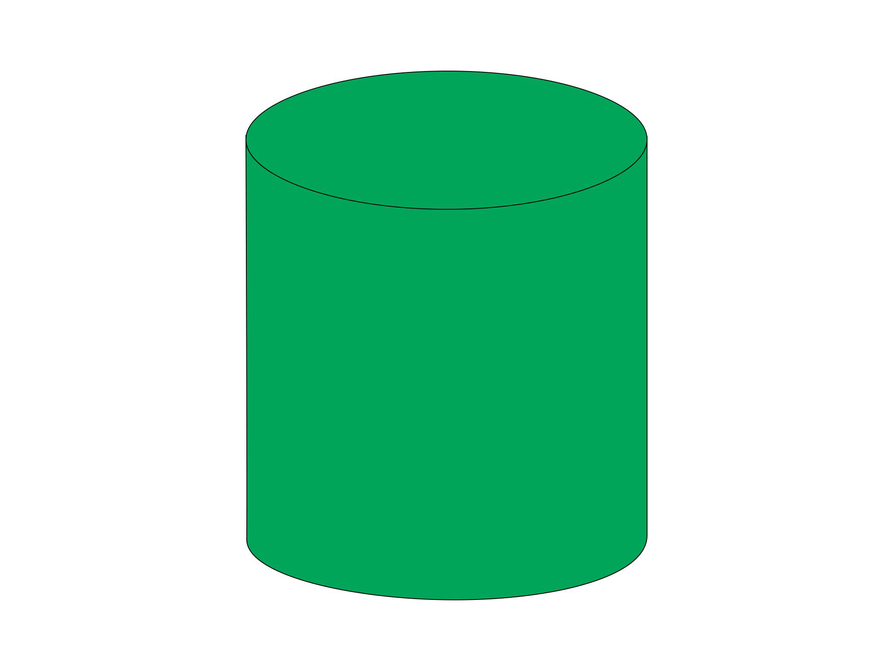# Project Work Time

Students should allocate their project work time time based upon need. Students might be at different stages of completion with their project. Student tasks may vary.

# Project Work Time

You may use one Gallery day to complete your project and/or to get help. * Think about the tasks you need to complete and organize your time.

# Cube Volume and Nets

2. For the figure below, the net is shown. Students will find that sometimes two surfaces might occupy the same space and will need to be connected to another edge.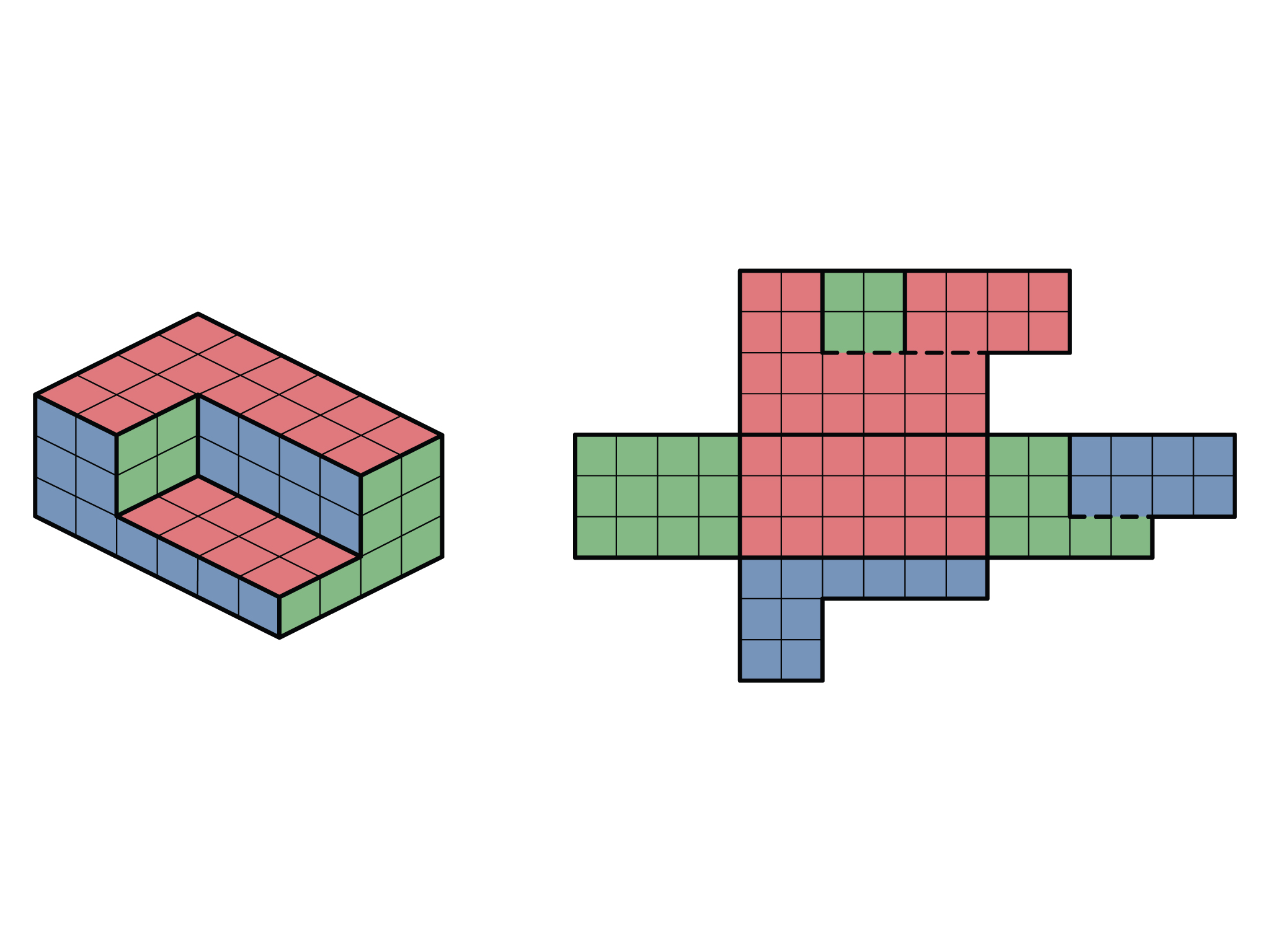3. Answers will vary, but the model should fold correctly and match what students have constructed using the tool.
4. Answers will vary, but if the net is drawn correctly, finding the surface area will be a matter of counting up the squares. Volumes will vary.

# Cube Volume and Nets

1. Create a solid made of snap cubes.
2. Make a net for the figure. This will take some experimenting and planning. Drawing the 2-D views may be helpful in visualizing the net. Think about where sides of the figure would share an edge.
3. Construct a model of the figure using the net.
4. Find the surface area and volume.

HANDOUT: Cube Volumes and Nets

# Same Surface Area, Different Volume

It may be helpful for students to work in pairs when they create two different figures with the same surface area but different volumes.

1. Answers will vary, but students might start with a rectangular prism and remove as many cubes as possible, while still keeping the same 2-D views. Students should see that the key is keeping the 2-D views the same. The larger a volume they start with, the more cubes can be removed. Here is a simple example: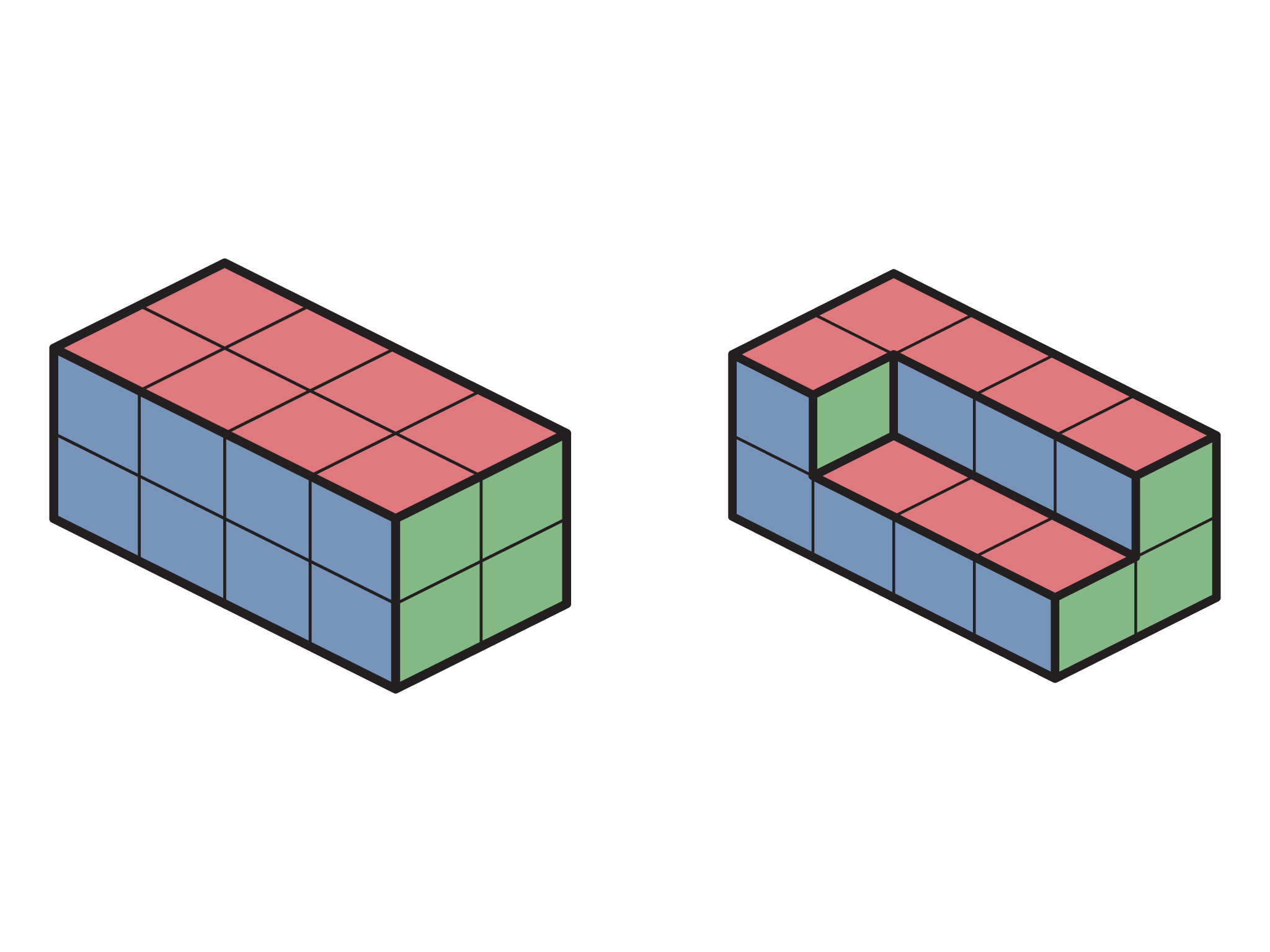2. Answers will vary. Here are the views for the example above: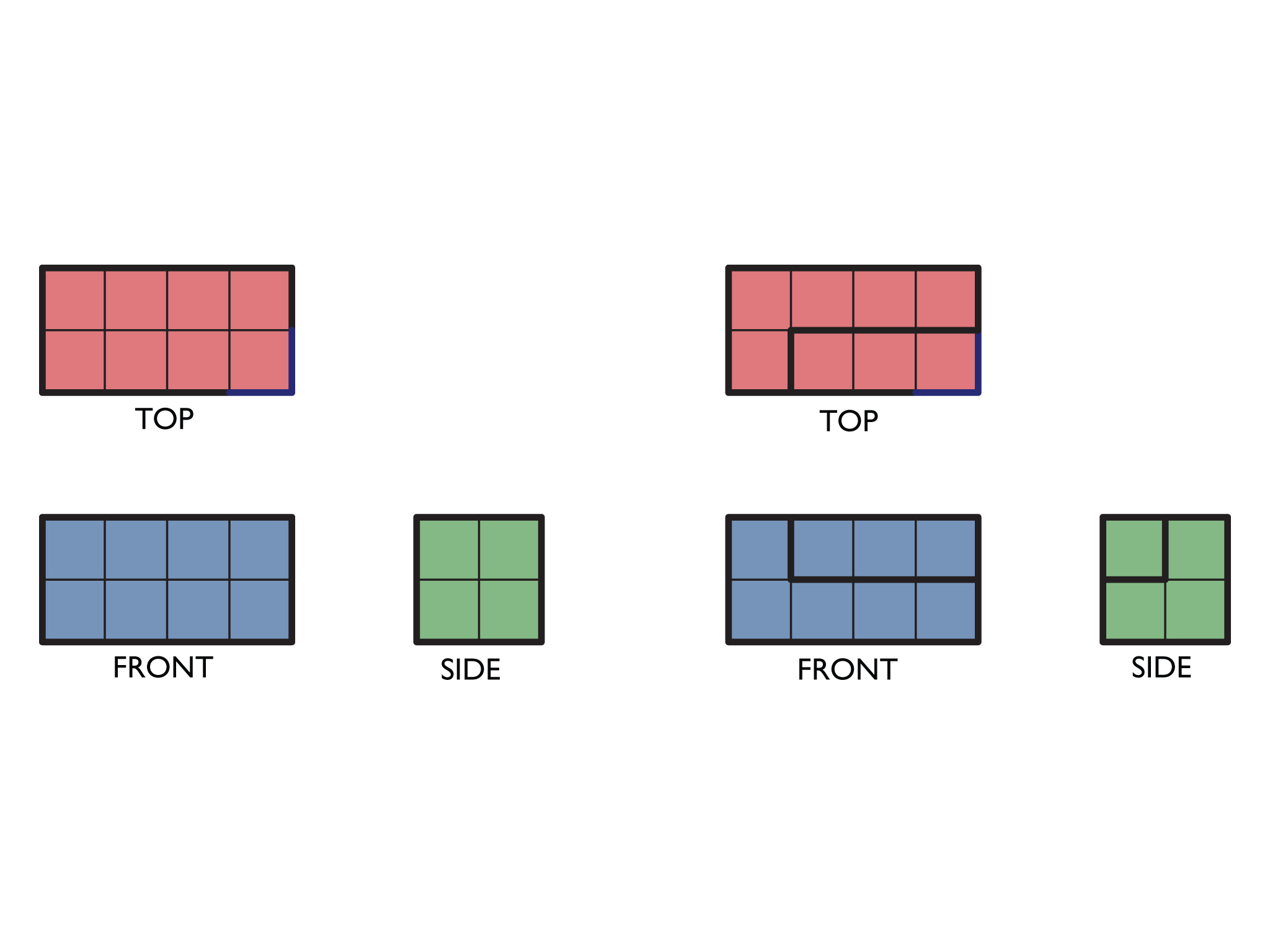3. Answers will vary, but it should be a matter of counting the number of cubes in each layer or finding the volume of the prism and subtracting the missing cubes.

# Same Surface Area, Different Volume

1. Create two solids that have the same surface area but different volumes. Make the volumes as different as possible.
2. Draw the 2-D views of each figure to show that the surface area is the same.
3. Find the volume of each figure.

# Tree House 2

This task gives students further practice making a scale drawing and thinking about the net of a solid. Students should also realize that the plans for a building are the 2-D views of the building and are similar to a net.

2. Answers will vary, but students should see that the bottom edge of each wall is shared with the sides of the floor. The net might look something like this: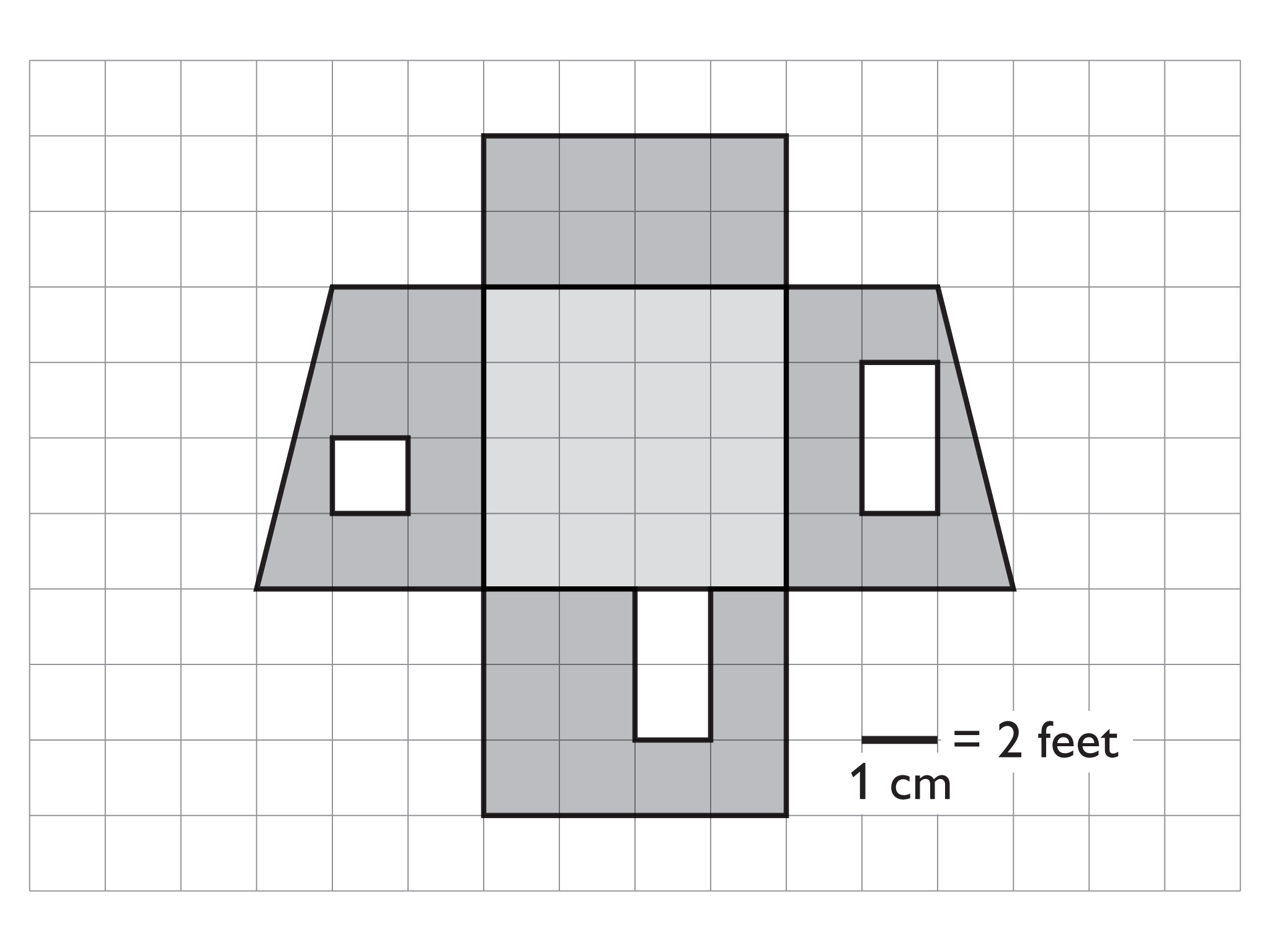3. Answers will vary. The sample above has a lateral surface area equal to 140 ft2 (160 ft2 minus 20 ft2 for the doors and windows).

4. Answers will vary. The volume of the sample above is 320 ft3. It can be found by breaking the shape into a rectangular prism and a triangular prism:

Rectangular prism volume: 8 • 8 • 4 = 256 ft3

Triangular prism volume: $\frac{1}{2}\left(8\cdot 2\right)\cdot 8=$ 64 ft3

Total volume: 256 ft3 + 64 ft3 = 320 ft3

# Tree House 2

1. Draw plans for a simple tree house with a flat roof. You can use the centimeter grid layout.
2. Make a net to construct a model of the tree house. Think about how the side views would be arranged to make a “net” (picture a box without a lid opening). The roof will be cut out separately since it will be a little larger than the floor area to overhang.
3. Find the lateral surface area.
4. Find the volume of the tree house.